Author: Oscar Cronquist Article last updated on August 01, 2022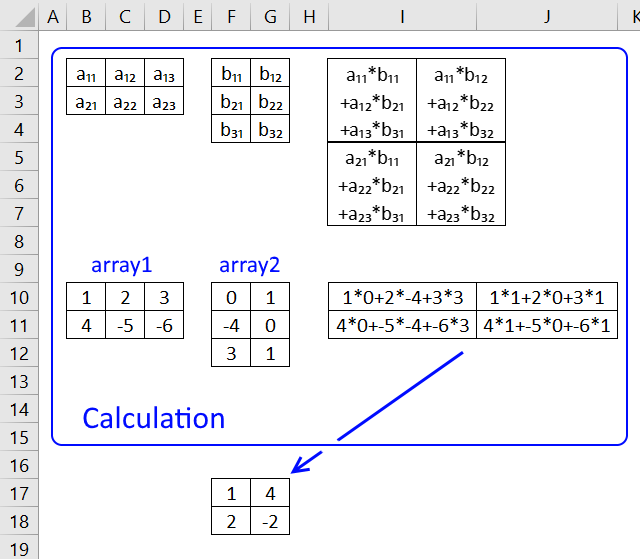The MMULT function calculates the matrix product of two arrays, an array as the same number of rows as array1 and columns as array2.

## 1. MMULT Function Syntax

MMULT(array1, array2)

## 2. MMULT Function Arguments

 array1 Required. The first array of numbers you want to multiply. array2 Required. The second array of numbers you want to multiply.

"For matrix multiplication, the number of columns in the first matrix must be equal to the number of rows in the second matrix. The resulting matrix has the number of rows of the first and the number of columns of the second matrix." Source: Wikipedia

This function must be entered as an array formula.

## 3. Spilled array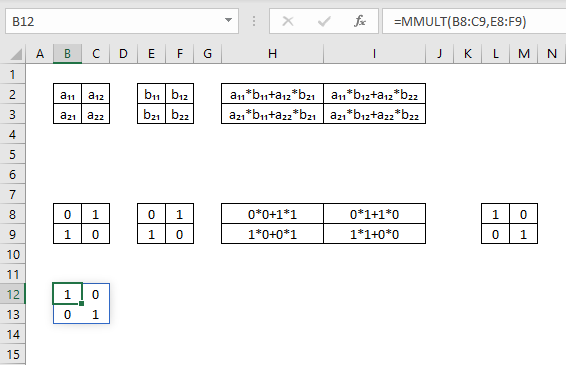Microsoft Excel has recently released an update to Excel 365 subscribers which allows you to use a new feature called dynamic array that replaces a regular array formula.

The image above shows the MMULT function in cell B12, however, it has been entered as a regular formula. Excel "knows" that it returns multiple values and automatically expands the range.

This is called "spilled array behavior" meaning the formula expands across cells automatically in order to show all values.

Note that only Excel 365 subscribers can use dynamic arrays.

There is a blue border around the values from a spilled array but it will disappear as soon as you press with left mouse button on a cell outside the blue border. It reappears again if you press with left mouse button on any cell in the spilled array.

The following examples demonstrate how the function works.

## 4. Cell ranges with an equal number of rows and columns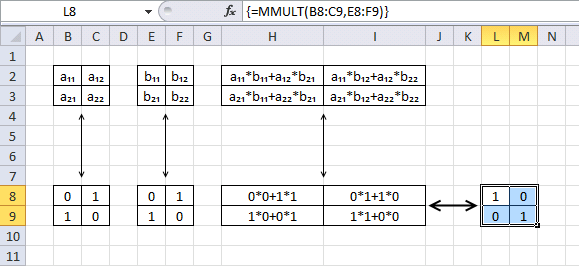Array1 (B2:C3) has 2 rows and array2 (E2:F3) has 2 columns, the returning array has 2 rows and 2 columns. Cell range B2:C3 and E2:F3 show the cell labels.

Cell range H2:I3 shows the calculations for each value in the array using the labels in B2:C3 and E2:F3.

Array formula in cell range L8:M9:

=MMULT(B8:C9,E8:F9)

Cell L8: a11*b11+a12*b21 becomes 0*0+1*1 and returns 1.

Cell L9: a21*b11+a22*b21 becomes 1*0+0*1 and returns 0.

Cell M8: a11*b12+a12*b22 becomes 0*1+1*0 and returns 0.

Cell M9: a21*b12+a22*b22 becomes 1*1+0*0 and returns 1.

## 5. Cell ranges with different numbers of rows and columns, example 1

Array1 (B2:C4) has 3 rows and array2 (E2:G3) has 3 columns, the returning array has 3 rows and 3 columns.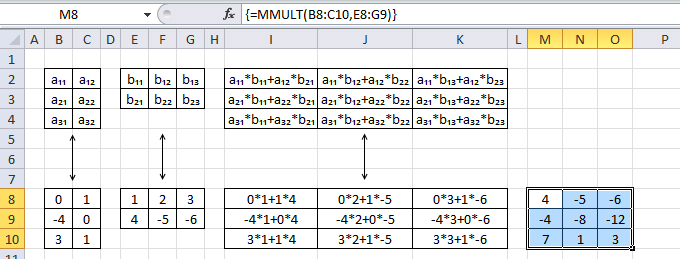Array formula in cell range L8:M9:

=MMULT(B8:C10,E8:G9)

Cell M8: a₁₁*b₁₁+a₁₂*b₂₁ becomes 0*1+1*4 and returns 4

Cell M9: a₂₁*b₁₁+a₂₂*b₂₁ becomes -4*1+0*4 and returns -4

Cell M10: a₃₁*b₁₁+a₃₂*b₂₁ becomes 3*1+1*4 and returns 7

Cell N8: a₁₁*b₁₂+a₁₂*b₂₂ becomes 0*2+1*-5 and returns -5

Cell N9: a₂₁*b₁₂+a₂₂*b₂₂ becomes -4*2+0*-5 and returns -8

Cell N10: a₃₁*b₁₂+a₃₂*b₂₂ becomes 3*2+1*-5 and returns 1

Cell O8: a₁₁*b₁₃+a₁₂*b₂₃ becomes 0*3+1*-6 and returns -6

Cell O9: a₂₁*b₁₃+a₂₂*b₂₃ becomes -4*3+0*-6 and returns -12

Cell O10: a₃₁*b₁₃+a₃₂*b₂₃ becomes 3*3+1*-6 and returns 3

This article shows you how to find a set of numbers that adds up to a sum you specify:

Recommended articles

Find numbers closest to sum
Excelxor is such a great website for inspiration, I am really impressed by this post Which numbers add up to […]

## 6. Cell ranges with different numbers of rows and columns, example 2

Array1 (B2:D3) has 2 rows and array2 (F2:G4) has 2 columns, the returning array has 2 rows and 2 columns.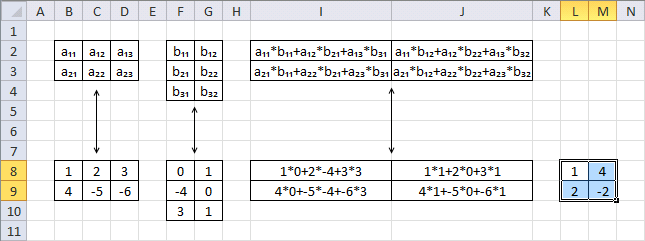Array formula in cell range L8:M9:

=MMULT(B8:D9,F8:G10)

Cell L8: a₁₁*b₁₁+a₁₂*b₂₁+a₁₃*b₃₁ becomes 1*0+2*-4+3*3 and returns 1

Cell L9: a₂₁*b₁₁+a₂₂*b₂₁+a₂₃*b₃₁ becomes 4*0+-5*-4+-6*3 and returns 2

Cell M8: a₁₁*b₁₂+a₁₂*b₂₂+a₁₃*b₃₂ becomes 1*1+2*0+3*1 and returns 4

Cell M9: a₂₁*b₁₂+a₂₂*b₂₂+a₂₃*b₃₂ becomes 4*1+-5*0+-6*1 and returns -2

## 7. Calculate totals row-wise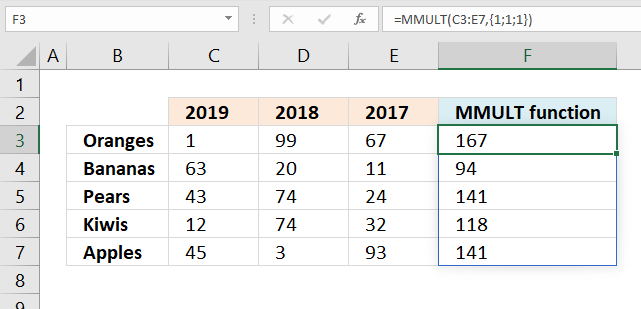The image above demonstrates an array formula that returns an array containing the totals from each row in cell range C3:E7.

=MMULT(C3:E7, {1; 1; 1})

The number of columns in the first cell range or array must be equal to the number of rows in the second cell range or array.

Cell range C3:E7 has 3 columns and array {1; 1; 1} has three rows. The resulting array has the same number of rows as the cell range and the same number of columns as the array, in this case the array has 5 rows and 1 column. To be able to add all numbers in I use value 1 in the array.

You can also create an array that grows automatically based on the cell range, named range or an Excel defined Table.

This formula demonstrates how to create the array in the second argument using a cell range:

=MMULT(C3:E7, TRANSPOSE(COLUMN(C3:E7)^0))

Let me explain this formula, the COLUMN function returns the corresponding column numbers from a cell range.

COLUMN(C3:E7) returns {3, 4, 5}.

COLUMN(C3:E7)^0

becomes

{3, 4, 5}^0

and returns {1, 1, 1}. Any number (except zero) to the power of 0 (zero) returns 1. This array has values distributed across columns, we need them transposed row-wise.

TRANSPOSE(COLUMN(C3:E7)^0)

becomes

TRANSPOSE({1, 1, 1}) and returns {1; 1; 1}.

This formula demonstrates how to create the array using a named range:

=MMULT(named_range, TRANSPOSE(COLUMN(named_range)^0))

This formula demonstrates how to create the array using a reference to an Excel Table:

=MMULT(Table1[A:C], TRANSPOSE(COLUMN(Table1[A:C])^0))

If you are an Excel 365 subscriber you can use the newly added SEQUENCE function:

=MMULT(C3:E7, SEQUENCE(COLUMNS(C3:E7))^0)

This post demonstrates how to refresh a scoreboard instantly using the MMULT function:

Recommended articles

Dynamic scoreboard
This article demonstrates a scoreboard, displayed to the left, that sorts contestants based on total scores and refreshes instantly each […]

## 8. Calculate totals column-wise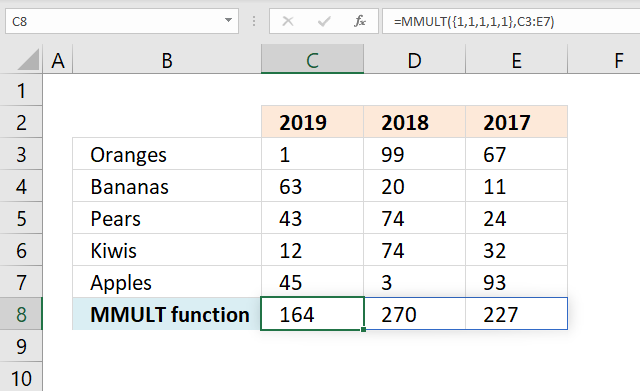This formula adds numbers and returns sums for each column in one calculation.

=MMULT({1, 1, 1, 1, 1}, C3:E7)

This article demonstrates how to search a data set with multiple strings using the MMULT function:

Recommended articles

Search each column for a string each and return multiple records – OR logic
RU asks: Can you please suggest if i want to find out the rows with fixed value in "First Name" […]